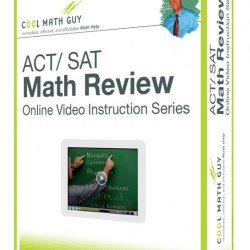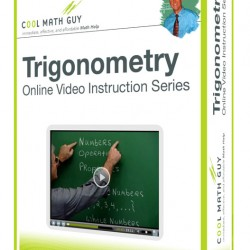# Courses

## Paid Courses## ACT / SAT Math Review

43 Lessons

The math review program contains over 15 hours of information designed to prepare students for the ACT or SAT standardized tests by (1) reviewing content contained on the tests and (2) working many problems written in the style of standardized tests. Emphasis is on test-taking strategies (much different from classroom strategies) to save time and reduce test anxiety. See the table of contents for more specific topic information.## Trigonometry

45 Lessons

Following a review of foundational algebra topics (primarily functions and the related topics of graphs, transformations, combinations, and inverses) the great power of Trigonometry in a “mathematical toolbox” is unveiled as the course continues into degree and radian measure, trig ratios, graphs of trig functions, trigonometric identities and equations, and vectors. Exponential and logarithmic functions are included, along with the study of conics, parametric equations, and polar coordinates. Use of the graphing calculator is emphasized.## College Algebra

52 Lessons

Topics include the study of functions extends to higher degree functions, transformations of graphs, and inverse functions. The study of quadratic functions in Algebra II extends to polynomial functions of higher degree and transformations of graphs. The study of matrices extends to operations with matrices and applications. Exponential and logarithmic functions as well as the study of conics are included. Use of the graphing calculator is emphasized.